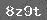# 第九册综合能力训练1

0.56÷7=

1.2×5=

1.9÷0.19=

7.5×4=

0.36÷18=

1.4×0.6=

2.1×0.3÷2.1

2.30÷8.1=

1.371.37÷1.37=

(1)80kg=(　　　 )t

(2)2h15min=(　　　 )h

(3)708dm2=(　　　 )m2

(4)比较3.72×0.43.72÷0.4的结果，(　　 )的得数比较大。

(5)200÷11的商用循环小数表示是(　　　 )，用四舍五入法保留两位小数约是(　　 　)(7)5.25÷2.5×4应先算(　　　 )法，后算(　　　 )法。

(1)5.25+5.75÷2.5×0.52f

    下一页

### 相关推荐

 留言与评论（共有 0 条评论）

 验证码：